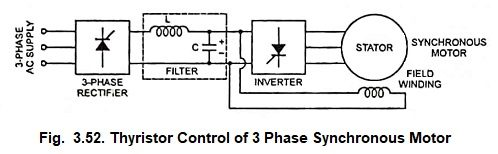## Thyristor Control of 3 Phase Synchronous Motor:

The synchronous motor is a constant speed motor and it devel­ops torque only at synchronous speed which is directly proportional to supply frequency. Therefore, variation of frequency of ac supply is a convenient method to control the speed of a syn­chronous motor. Before the advent of power electronic devices change of frequency was a very difficult task. However, the power electronic circuits provide a simple method of changing the frequency. The 50 Hz ac is converted into dc by a controlled rectifier.

A variable frequency inverter converts dc into variable frequency ac. To keep the flux density in the motor constant voltage and frequency must be varied in the same ratio so that voltage/frequency ratio is constant.### Working Principle:

Figure 3.52 shows a system of thyristor control of 3 phase synchronous motor. The 3-phase 50 Hz ac is rectified by a controlled 3-phase rectifier. The filter removes harmonics from the output of the rectifier. The variable frequency inverter feeds a variable frequency ac to the synchronous motor. Thus the speed of a synchronous motor can be controlled. The dc output from filter is also used to excite the rotor winding.

In low-speed high power applications it is also possible to use a cycloconverter to convert 50 Hz ac into variable frequency ac. The cycloconverter does the conversion without an intermediate dc link. The maximum output frequency is limited to about one-third of the line frequency so that the output waveform has low harmonic content.

Scroll to Top